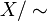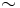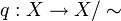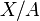# Quotient topology

## Definition

### Quotient topology by an equivalence relation

Suppose$X$ is a topological space and$\! \sim$ is an equivalence relation on$X$. In other words,$\! \sim$ partitions$X$ into disjoint subsets, namely the equivalence classes under it. The quotient space of$X$ by$\! \sim$, or the quotient topology of$X$ by$\! \sim$, denoted$\! X/\sim$, is defined as follows:

• As a set, it is the set of equivalence classes under$\sim$.
• The topology on it is defined as the finest topology possible so that the quotient map$\! q:X \to X/\sim$, that sends every element$x \in X$ to its equivalence class, is a continuous map. Equivalently, the open sets in the topology on$\! X/\sim$ are those subsets of$\! X/\sim$ whose inverse image in$X$ (which is the union of all the corresponding equivalence classes) is an open subset of$X$.

The map$q:X \to X/\sim$ is a quotient map. In fact, the notion of quotient topology is equivalent to the notion of quotient map (somewhat similar to the first isomorphism theorem in group theory?)

### Quotient topology by a subset

Suppose$X$ is a topological space and$A$ is a subset of$X$. The quotient space$X/A$ is defined as the quotient space$\! X/\sim$, where$\sim$ is the equivalence relation that identifies all points of$A$ with each other but not with any point outside$A$, and does not identify any distinct points outside$A$. In other words, all points of$A$ become one equivalence class, and each single point outside$A$ forms its own equivalence class.

Note that a notation of the form$X/A$ should be interpreted carefully. In case$X$ is a topological group and$A$ is a subgroup, this notation is to be intepreted as the coset space, and not in terms of the description given above. Context is extremely important.

### Quotient topology by a subset with based topological space interpretation

Suppose$X$ is a topological space and$A$ is a subset of$X$. We may be interested in the pair of topological spaces$(X,A)$. In this context,$X/A$ (as defined above) is often viewed as a based topological space, with basepoint chosen as the equivalence class of$A$.